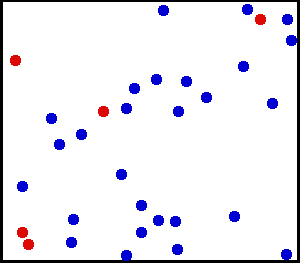## The Effect of the Finite Volume

#### Learning Objective

• Demonstrate an understanding of the van der Waals equation for non-ideal gases.

#### Key Points

• The ideal gas law assumes that gases are composed of point masses that interact via completely elastic collisions.
• Real gases are made up of particles that occupy a non-zero volume known as the excluded volume.
• The van der Waals equation includes a volume-correction term that is specific to each gas; if a gas is behaving in an ideal manner, the correction term becomes negligible relative to the total volume.

#### Term

• excluded volumethe volume occupied by non-ideal gas particles

The ideal gas law is commonly used to model the behavior of gas-phase reactions. Ideal gases are assumed to be composed of point masses whose interactions are restricted to perfectly elastic collisions; in other words, a gas particles’ volume is considered negligible compared to the container’s total volume.Gas particlesIdeal gases are assumed to be composed of point masses that interact via elastic collisions.

There are two notable situations in which the behavior of real gases deviates from that model:

1. At high pressures where the volume occupied by gas molecules does not approach zero
2. At low temperatures where the contribution of intermolecular forces becomes significant

## Excluded Volume and the van der Waals Equation

The particles of a real gas do, in fact, occupy a finite, measurable volume. At high pressures, the deviation from ideal behavior occurs because the finite volume that the gas molecules occupy is significant compared to the total volume of the container. The van der Waals equation modifies the ideal gas law to correct for this excluded volume, and is written as follows:

$P(V - nb) = nRT$

The available volume is now represented as $V - nb$, where b is a constant that is specific to each gas. In this approximation, the gas molecules are considered hard spheres with a defined radius (r) that cannot overlap with the radius of a neighboring particle. The constant b is defined as:

$b = 4N_A \cdot \frac{4}{3} \pi \cdot r^{3}$

where NA is Avogadro’s number and r is the radius of the molecule.

It is important to note that this equation applies to ideal gases as well. It can be simplified because in an ideal situation, the value of b is so much smaller than V that it does not make a measurable difference in the calculation.Translational motion of heliumUnder certain conditions, such as high pressure, real gases do not always behave according to the ideal model. Here, the size of helium atoms relative to their spacing is shown to scale under 1,950 atmospheres of pressure.